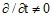# Kinematic models in Flux

## Introduction

This paragraph deals with the main kinematic models available in Flux: “multi-static” model, “imposed speed” model, “coupled load” model.

These three models are briefly described below and illustrated with examples.

There are also two other kinematic models that are used: imposed position and multiphysics position that are not described in this document.

## Multi-static kinematic model

In the multi-static kinematic model, the moving part of the device is not moving.

The computation of the electromagnetic field is carried out for various arbitrary relative positions of moving and fixed parts. This model performs a set of Magneto Static* computations and does not take into consideration the dynamics equation. This model is equivalent to a parametrized study where the position of the moving part is a varying parameter.

Note: *This model can also be used with the Steady state AC Magnetic applications.

## Imposed speed kinematic model

In the imposed speed kinematic model, the moving part is considered as moving at a constant velocity with respect to the fixed part.

The computation of the electromagnetic field is carried out for the different positions defined by the imposed speed of the moving part. As in the previous kinematic model (multi-static), the dynamics equation is not considered.

In the imposed speed kinematic model, the physical application used is the Transient Magnetic physical application. In this case, the Maxwell's equations consider the time dependence of the electromagnetic field ().

## Important notes

The imposed speed model and the multi-static model offer the same results if the sources of the electromagnetic field are constant: constant current sources, permanent magnets, … and that there is no coupling circuit

On the other hand, the results of these two models are different under the following conditions:

• time varying sources: varying current sources (directly in the Flux project or through a circuit coupling), …
• conductive regions, where eddy currents occur, …